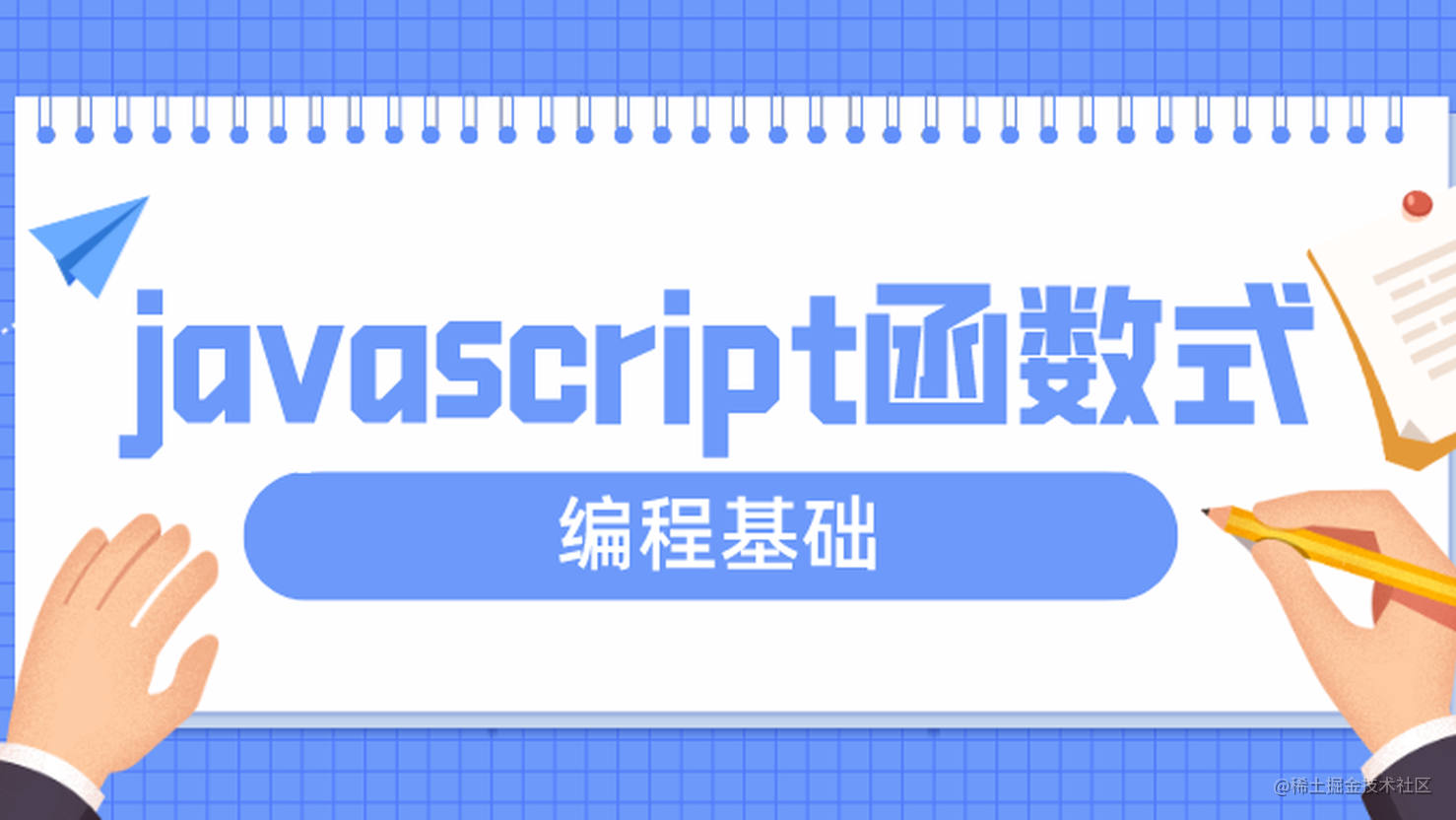# 三、纯函数（函数式编程的基石，无副作用的函数）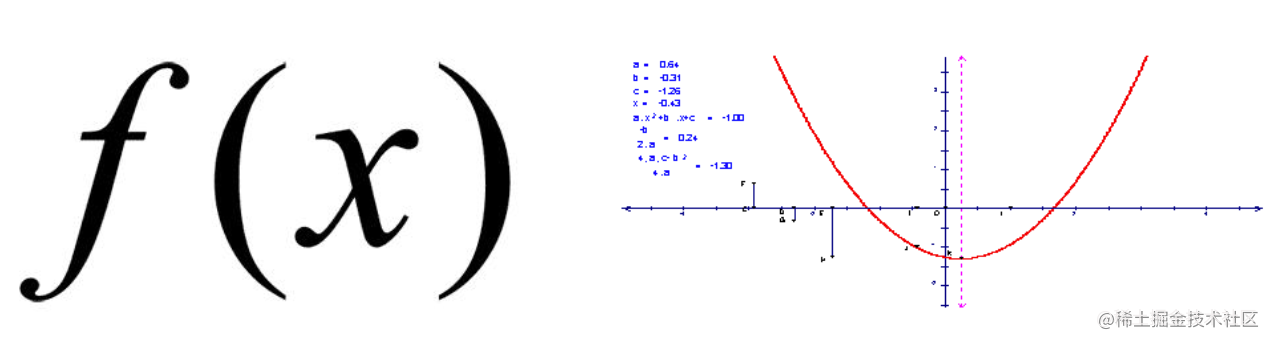在初中数学里，函数 f 的定义是：对于输入 x 产生一个唯一输出 y=f(x)。这便是纯函数。它符合两个条件：1.此函数在相同的输入值时，总是产生相同的输出。函数的输出和当前运行环境的上下文状态无关。2.此函数运行过程不影响运行环境，也就是无副作用（如触发事件、发起 http 请求、打印/log 等）。简单来说，也就是当一个函数的输出不受外部环境影响，同时也不影响外部环境时，该函数就是纯函数，也就是它只关注逻辑运算和数学运算，同一个输入总得到同一个输出。javascript 内置函数有不少纯函数，也有不少非纯函数。纯函数：Array.prototype.sliceArray.prototype.mapString.prototype.toUpperCase 非纯函数：Math.randomDate.nowArray.ptototype.splice 这里我们以 slice 和 splice 方法举例：

``````var xs = [1,2,3,4,5];
// 纯的
xs.slice(0,3);
//=> [1,2,3]
xs.slice(0,3);
//=> [1,2,3]
xs.slice(0,3);
//=> [1,2,3]

// 不纯的
xs.splice(0,3);
//=> [1,2,3]
xs.splice(0,3);
//=> [4,5]
xs.splice(0,3);
//=> []

``````var squareNumber  = memoize(function(x){ return x*x; });
squareNumber(4);
//=> 16
squareNumber(4); // 从缓存中读取输入值为 4 的结果
//=> 16

``````var minimum = 21;
var checkAge = function(age) {
return age >= minimum;
};

``````var checkAge = function(age) {
var minimum = 21;
return age >= minimum;
};

# 四、函数柯里化

curry 的概念很简单：将一个低阶函数转换为高阶函数的过程就叫柯里化。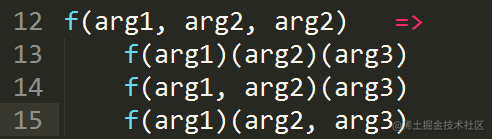用一个形象的比喻就是：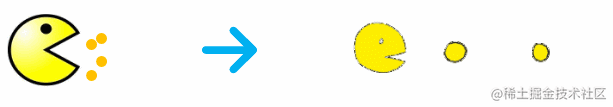比如对于加法操作：var add = (x, y) => x + y，我们可以这样柯里化：

``````//es5写法
return function(y) {
return x + y;
};
};

//es6写法
var add = x => (y => x + y);

//试试看

increment(2);  // 3

``````var checkage = min => (age => age > min);
var checkage18 = checkage(18);
checkage18(20);
// =>true

``````var curry = require('lodash').curry;

//柯里化两个纯函数
var match = curry((what, str) => str.match(what));
var filter = curry((f, ary) => ary.filter(f));

//判断字符串里有没有空格
var hasSpaces = match(/\s+/g);

hasSpaces("hello world");  // [ ' ' ]
hasSpaces("spaceless");  // null

var findSpaces = filter(hasSpaces);

findSpaces(["tori_spelling", "tori amos"]);  // ["tori amos"]

# 五、函数组合

``````var toUpperCase = function(x) { return x.toUpperCase(); };
var exclaim = function(x) { return x + '!'; };

var shout = function(x){
return exclaim(toUpperCase(x));
};

shout("send in the clowns");
//=> "SEND IN THE CLOWNS!"

``````//定义compose
var compose = (...args) => x => args.reduceRight((value, item) => item(value), x);

var toUpperCase = function(x) { return x.toUpperCase(); };
var exclaim = function(x) { return x + '!'; };

var shout = compose(exclaim, toUpperCase);

shout("send in the clowns");
//=> "SEND IN THE CLOWNS!"

``````var head = function(x) { return x; };
var reverse = reduce(function(acc, x){ return [x].concat(acc); }, []);

last(['jumpkick', 'roundhouse', 'uppercut']);
//=> 'uppercut'

# 六、声明式和命令式代码

``````// 命令式
var makes = [];
for (var i = 0; i < cars.length; i++) {
makes.push(cars[i].make);
}

// 声明式
var makes = cars.map(function(car){ return car.make; });

# 七、Point Free

pointfree 模式指的是，永远不必说出你的数据。它的意思是说，函数无须提及将要操作的数据是什么样的。一等公民的函数、柯里化（curry）以及组合协作起来非常有助于实现这种模式。

``````// 非 pointfree，因为提到了数据：word
var snakeCase = function (word) {
return word.toLowerCase().replace(/\s+/ig, '_');
};

// pointfree
var snakeCase = compose(replace(/\s+/ig, '_'), toLowerCase);

# 八、示例应用

2.向 flickr 发送 api 请求
3.把返回的 json 转为 html 图片
4.把图片放到屏幕上上面提到了两个不纯的动作，即从 flickr 的 api 获取数据和在屏幕上放置图片这两件事。我们先来定义这两个动作，这样就能隔离它们了。这里我们只是简单包装了一下 jQuery 的 getJSON 函数，把它变为一个 curry 函数，还有就是把参数位置也调换了下，我们把它们放在 Impure 命名空间下以用来隔离，这样我们就知道它们都是危险函数。运用函数柯里化和函数组合的技巧，我们就可以创建一个函数式的实际应用了：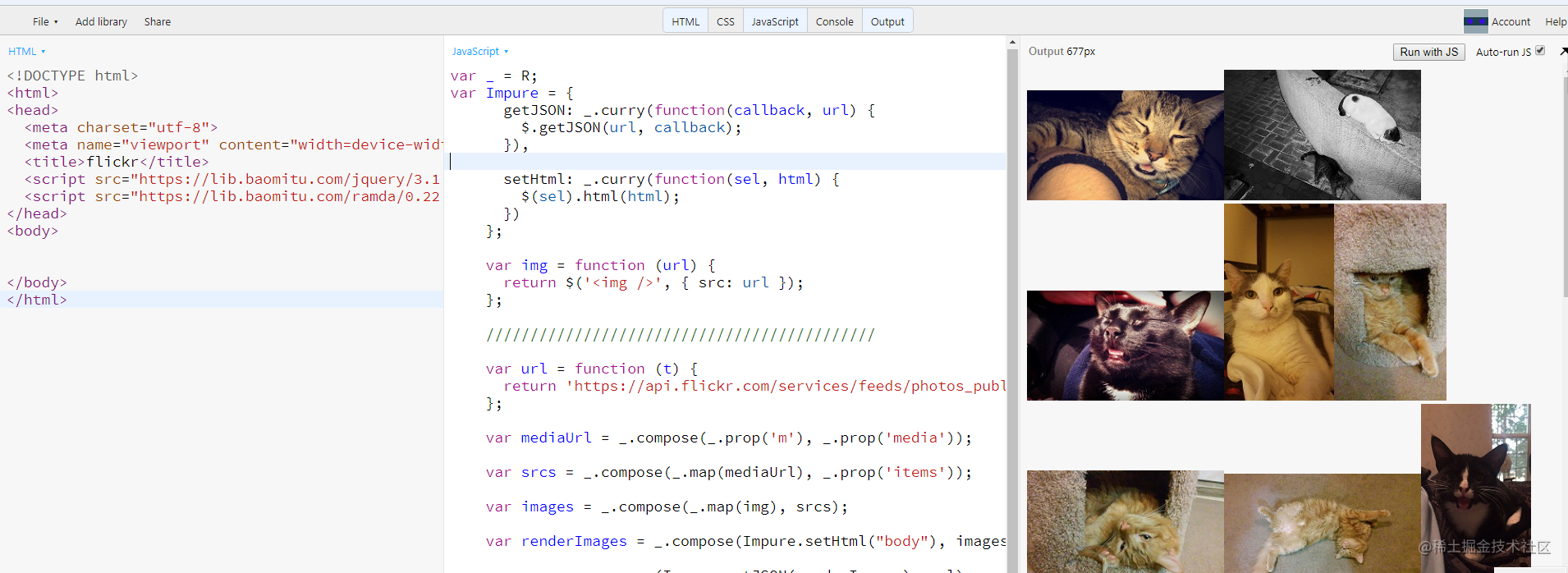预览地址：demo看看，多么美妙的声明式规范啊，只说做什么，不说怎么做。现在我们可以把每一行代码都视作一个等式，变量名所代表的属性就是等式的含义。